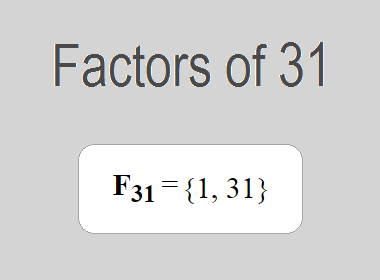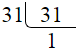# Factors of 31The factors of 31 are 1 and 31 i.e. F31 = {1, 31}. The factors of 31 are all the numbers that can divide 31 without leaving a remainder. 31 is a prime number, so it is divisible by 1 and 31 only.

We can check if these numbers are factors of 31 by dividing 31 by each of them. If the result is a whole number, then the number is a factor of 31. Let's do this for each of the numbers listed above:

·        1 is a factor of 31 because 31 divided by 1 is 31.

·        31 is a factor of 31 because 31 divided by 31 is 1.

## Properties of the Factors of 31

The factors of 31 have some interesting properties. One of the properties is that the sum of the factors of 31 is equal to 32. We can see this by adding all the factors of 31 together:

1 + 31 = 32

Another property of the factors of 31 is that the only prime factor of 31 is 31 itself.

********************

********************

## Applications of the Factors of 31

The factors of 31 have several applications in mathematics. One of the applications is in finding the highest common factor (HCF) of two or more numbers. The HCF is the largest factor that two or more numbers have in common. For example, to find the HCF of 31 and 62, we need to find the factors of both numbers and identify the largest factor they have in common. The factors of 31 are 1, and 31. The factors of 62 are 1, 2, 31, and 62. The largest factor that they have in common is 31. Therefore, the HCF of 31 and 62 is 31.

Another application of the factors of 31 is in prime factorization. Prime factorization is the process of expressing a number as the product of its prime factors. The prime factor of 31 is 31 since it is only the prime number that can divide 31 without leaving a remainder. Therefore, we can express 31 as:

31 = 31

We can do prime factorization by division method as given below,31 = 31

Since 31 is a prime number, there is no factor tree of 31.

## Conclusion

The factors of 31 are the numbers that can divide 31 without leaving a remainder. The factors of 31 are 1, and 31. The factors of 31 have some interesting properties, such as having a sum of 32. The factors of 31 have several applications in mathematics, such as finding the highest common factor and prime factorization.